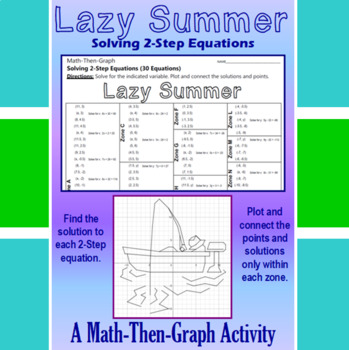# Lazy Summer - A Math-Then-Graph Activity - Solve 2-Step EquationsSubject
Resource Type
File Type

PDF

(786 KB|5 pages)
Also included in:
1. Enjoy Summer Time and give students the practice they need at solving 2-step equations with this Math-Then-Graph Activity. Students are given a list of coordinate points to plot. Some of the points are incomplete. The students need to solve for either x or y in a 2-step equation to complete the poin
\$15.75
\$9.00
Save \$6.75
Product Description

Enjoy Summer Time and give students the practice they need at solving 2-step equations with this Math-Then-Graph Activity.

Students are given a list of coordinate points to plot. Some of the points are incomplete. The students need to solve for either x or y in a 2-step equation to complete the point. The students should connect the completed coordinate points only within the designated zones. When they are done, they will have graphed a picture of a fisherman having a very Lazy Summer.

Here are some tips:

 All points will be on one grid line or another.

 A completed picture has been provided to be used as a key.

 I have also found that printing graph paper directly from themathworksheetsite.com

helps out, but any graph paper will do.

 Remember to copy double-sided when necessary to save paper

Here are some other Summer Time Math-Then-Graph Activities you may like:

Summer Shade - A Math-Then-Graph Activity - Solve 2-Step Equations

Summer Swim - A Math-Then-Graph Activity - Solve 2-Step Equations

Summer Escape - A Math-Then-Graph Activity - Solve 2-Step Equations

Summer Bonfire - A Math-Then-Graph Activity - Solve 2-Step Equations

Aloha Summer - A Math-Then-Graph Activity - Solve 2-Step Equations

A Summer Treat - A Math-Then-Graph Activity - Solve 2-Step Equations

Summer Camp - A Math-Then-Graph Activity - Solve 2-Step Equations

Crack of the Bat - A Math-Then-Graph Activity - Solve 2-Step Equations

Or purchase all 9 in one Bundle.

Summer Time Big Bundle - 9 Math-Then-Graph - Solve 2-Step Equations

Also, don't forget to visit my web site:

Mike's Math Market

to see my other Coordinate Graphing Activities

and Math-Then-Graph Activities at affordable prices.

Have Fun!

Total Pages
5 pages
Included
Teaching Duration
55 minutes
Report this Resource to TpT
Reported resources will be reviewed by our team. Report this resource to let us know if this resource violates TpT’s content guidelines.